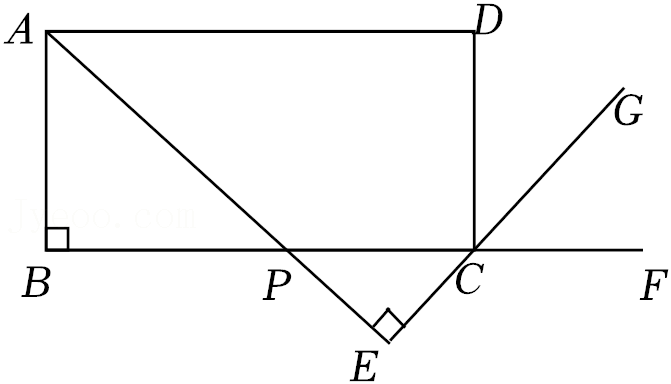(1) 若 $\mathrm{CG}$ 为 $\angle \mathrm{DCF}$ 的平分线. 请判断 $\mathrm{BP}$ 与 $\mathrm{CP}$ 的数量关系, 并 证明;
(2) 若 $\mathrm{AB}=3, \triangle \mathrm{ABP} \cong \triangle \mathrm{CEP}$, 求 $\mathrm{BP}$ 的长.